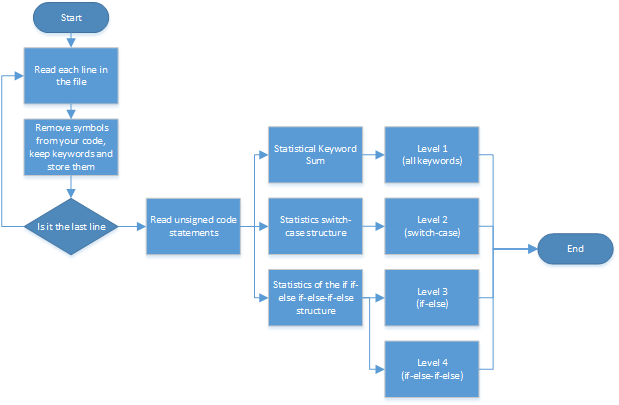# EE308 Lab2

 The Link Your Class https://bbs.csdn.net/forums/MUEE308FZU202201 The Link of Requirement of This Assignment https://bbs.csdn.net/topics/608745426 The Aim of This Assignment For independent programming, version control(git) MU STU ID and FZU STU ID 20124856_832001222

### 1.PSP form for this work.

 Process Stages Work Time Pre-work Read the requirements and install git 25min Design Design program execution flow 30min Coding Coding implementation process 30min Test The output of the test code 10min Commit Submit the code to GitHub 5min Final Sum up the experience 30min

### 2.Problem-solving ideas

2.How do I get the code in the file

3.How do I remove symbols from my code

4.Design data structures to hold statistical results

### 3.Implementation process.### 4.Code description

4.1 Read the code in the file and remove symbols

``````while line:
line = line[:-1]
line = line.replace("else if", "else-if")
x = re.split(' |\(|\;|\\t|\:|\{|\/\/', line)
for i in x:
for j in range(kwd_len):
if i == kwdlst[j]:
if i == "switch":
num0s.append(num0)
num0 = 0
if i == "case":
num0 += 1
count[j] += 1
for i in x:
if i == "if" or i == "else" or i == "else-if":
sentences.append(x)
continue
f.close()``````

4.2 Statistics the total number of all keywords & Switch-case

``````for i in range(kwd_len):
if count[i] != 0:
sumres += count[i]
if (i == 30):
sumres += count[i]``````

4.3 Statistics of the if if-else if-else-if-else structure

``````for j in range(x_len):
for i in sentences:
if i[j] == "if":
flag_if = 1
if i[j] == "else-if":
flag_else_if = 1
if i[j] == "else":
if flag_else_if == 1:
num_if_else_if_else += 1
elif flag_if == 1:
num_if_else += 1
flag_else_if = 0
flag_else = 0``````

### 5.Test results

"D:\Program Files\Python38\python.exe" D:\worksapce\lab1-2\Lab2.py
level of stastic (from 1 to 4): 4
sum :  35
switch  num :  2
case num [3, 2]
if-else num: 1
if-elseif-else num: 1

### 6.Summarize

In this assignment, I learned the combination of git and PyCharm and the importance of version control in code development, which laid a solid foundation for future code development.

...全文
76 回复 打赏 收藏 转发到动态 举报EE308 LAB2
EE308 LAB2LAB2 Progress LoggingⅠ. Use Git to conduct version control1. Create Github Repository2. Clone your Repo to local3. Make your first commit! LAB2 Progress Logging Ⅰ. Use Git to conduct version control 1. Create Github Repository To 2. Clone your ReEE308 Lab2 博饼小程序（JAVA）EE308 Lab1
EE308 Lab1

285发帖与我相关我的任务• 近7日
• 近30日
• 至今